# Rajasthan Board Question Paper for Class 10 Maths 2015 In PDF

## RBSE 10th Maths Question Papers 2015 With Solutions

Rajasthan Board Class 10th maths 2015 question paper, along with detailed solutions is uploaded here in a downloadable pdf format and also in the text. Along with the solutions, they can also get the RBSE 10th standard maths question paper 2015 for reference. Students are advised to refer all the Rajasthan board previous year maths question papers here. The papers uploaded on BYJU’S contain actual questions asked in the RBSE 10th Exam, and there is a chance of getting the same question in your question papers. Solving 2015 Maths question papers for class 10 will help the students to solve the different types of questions and strategize their preparation.

### QUESTION PAPER CODE S–09–Mathematics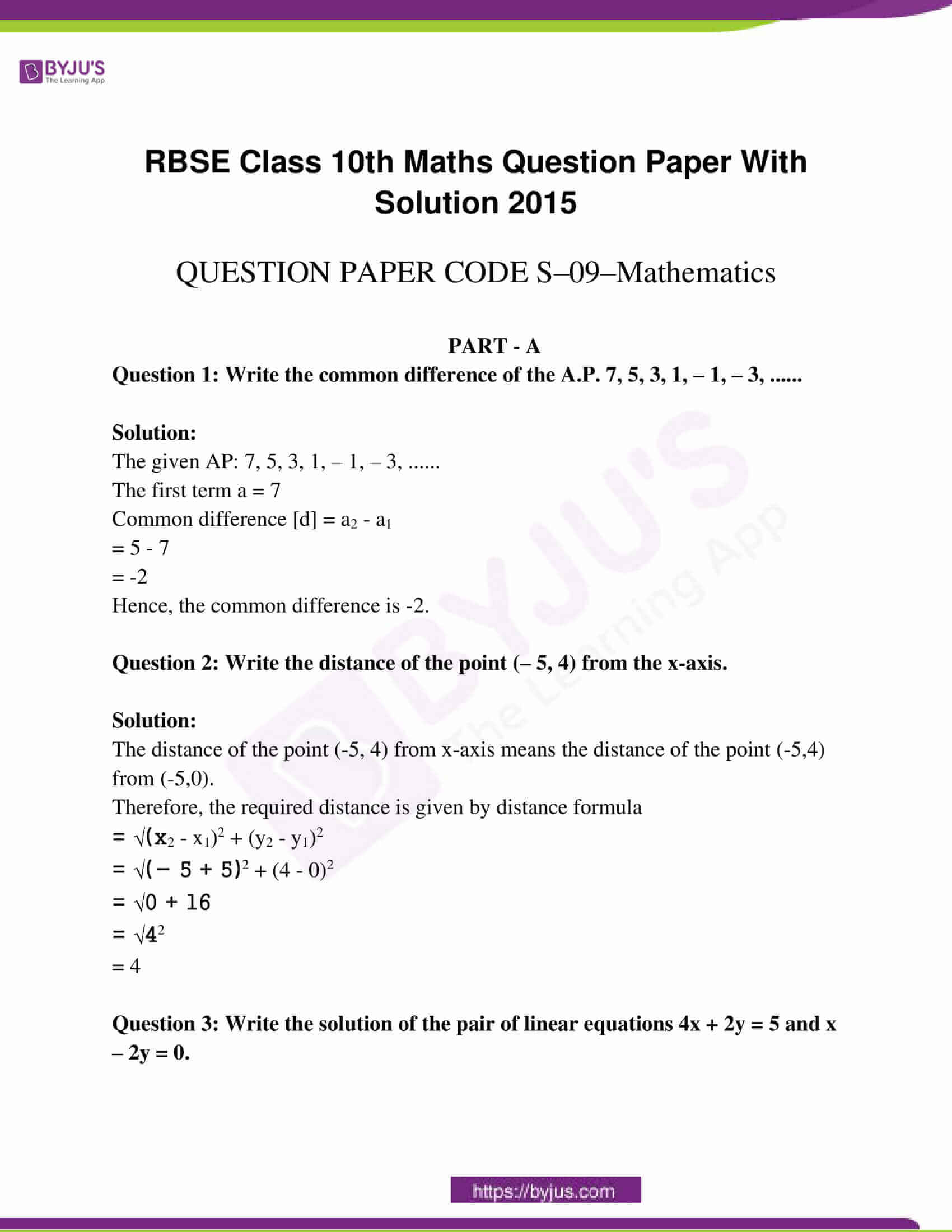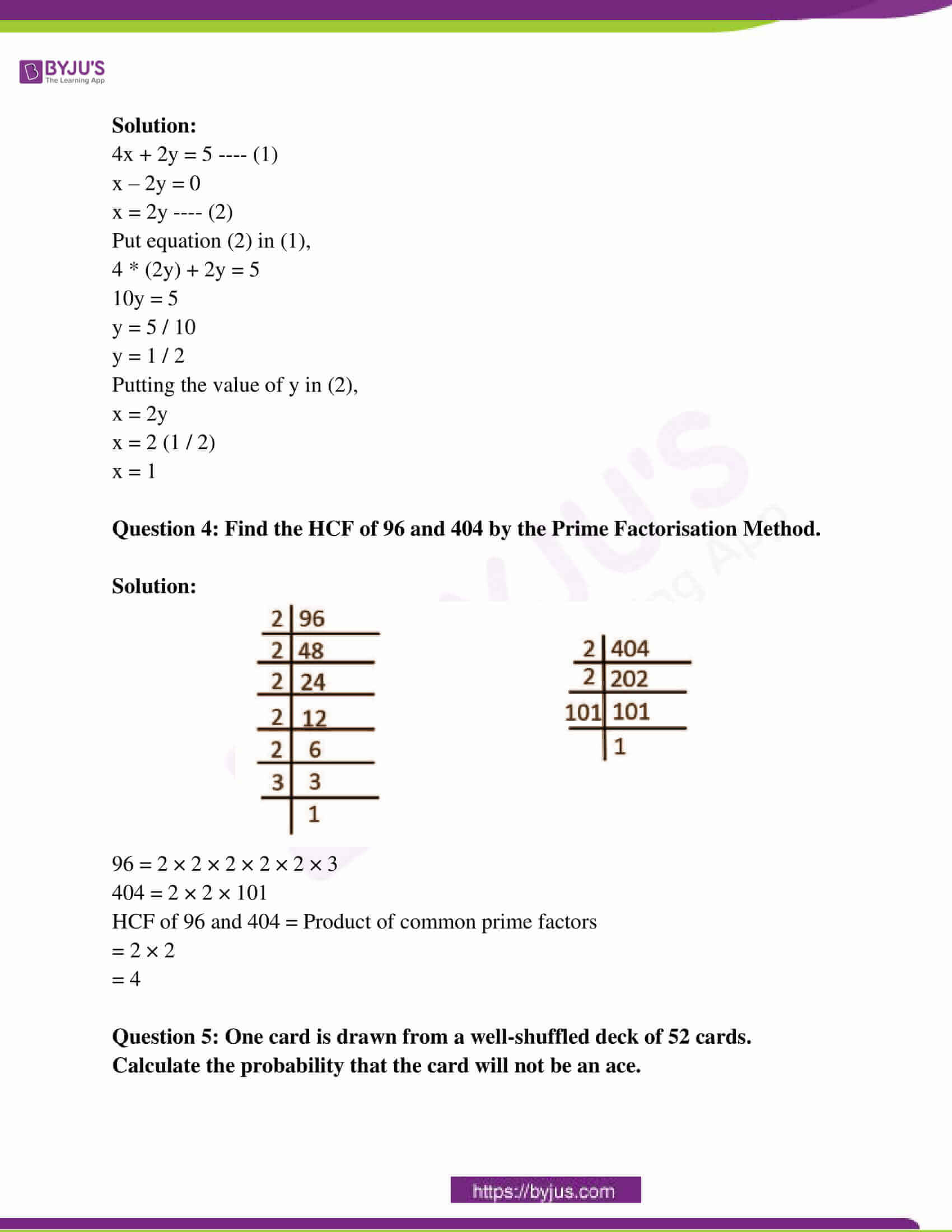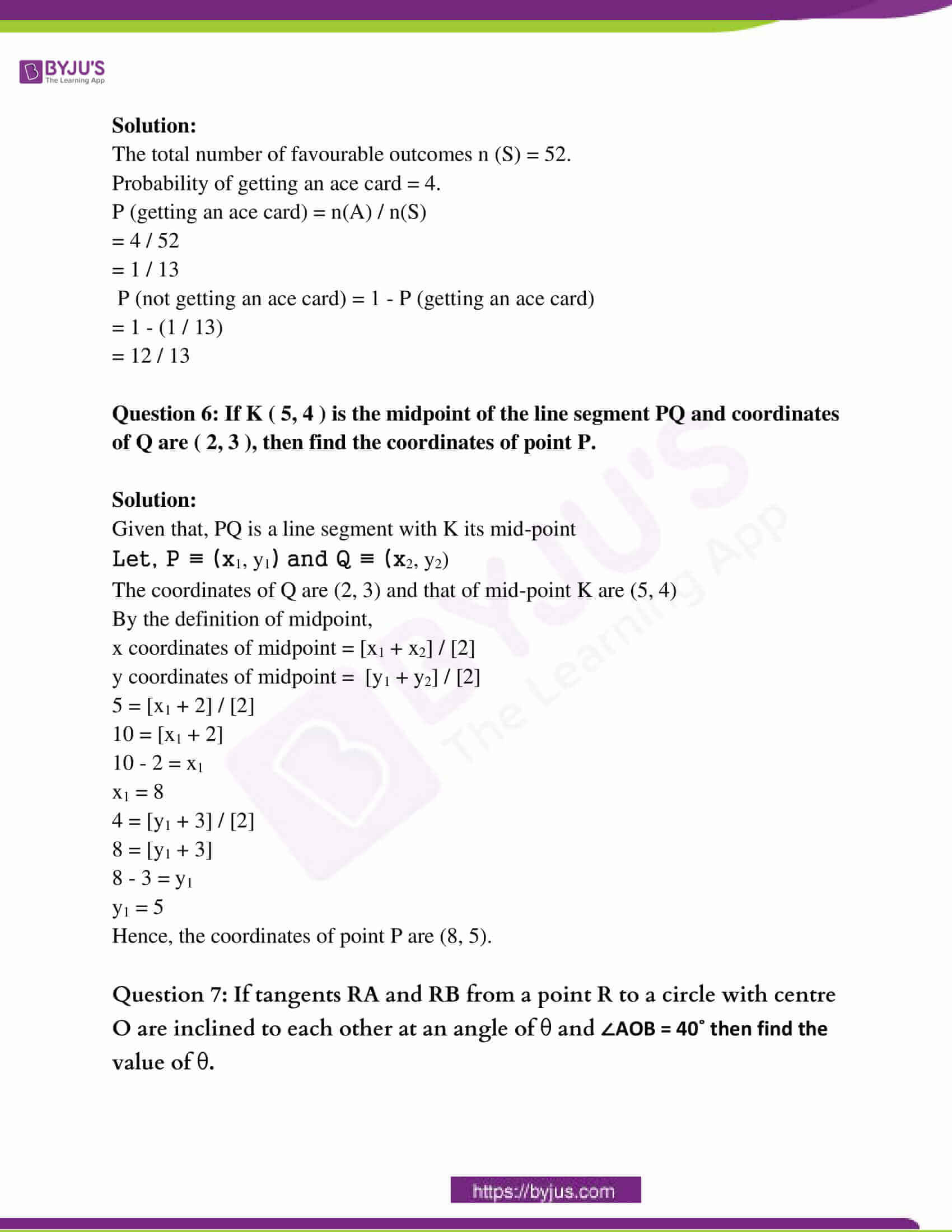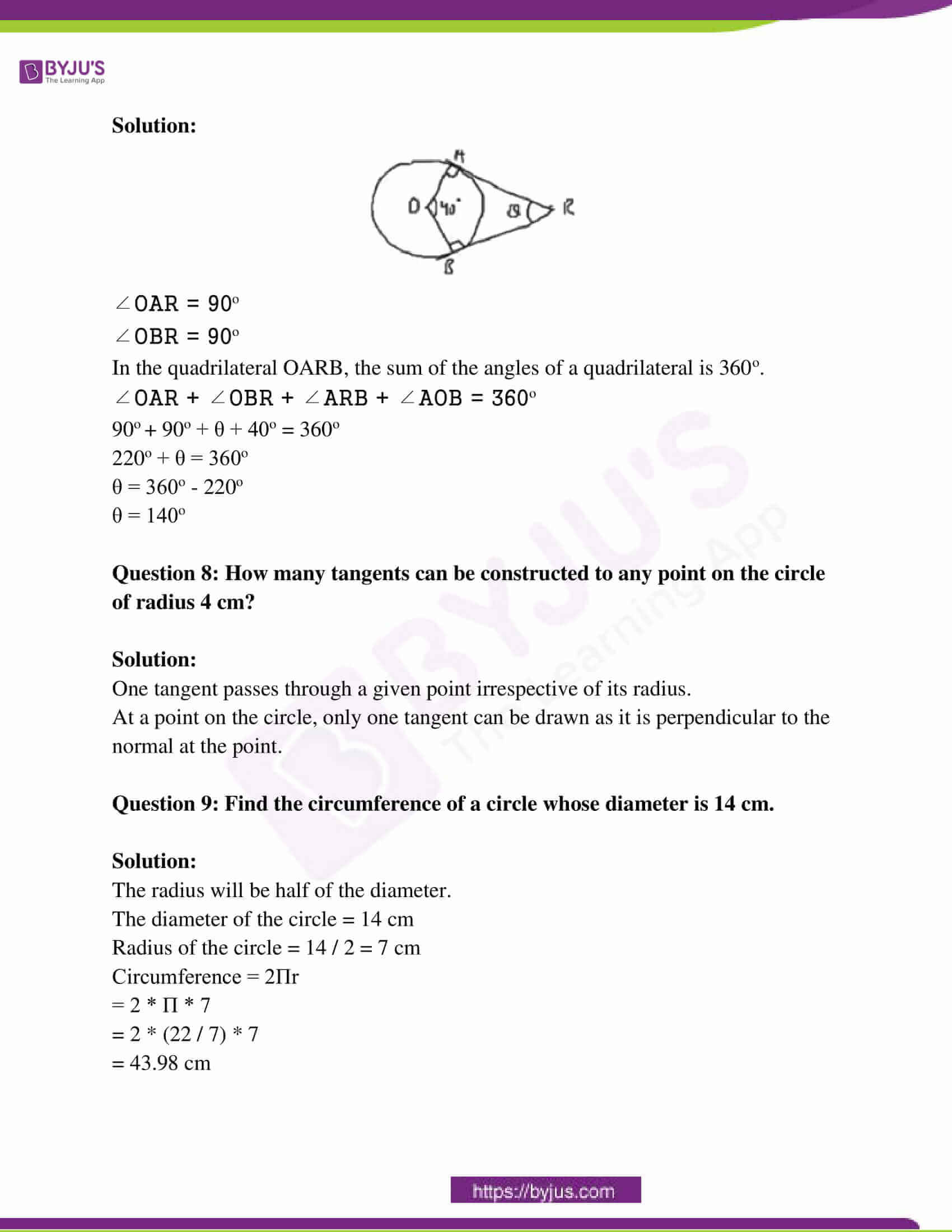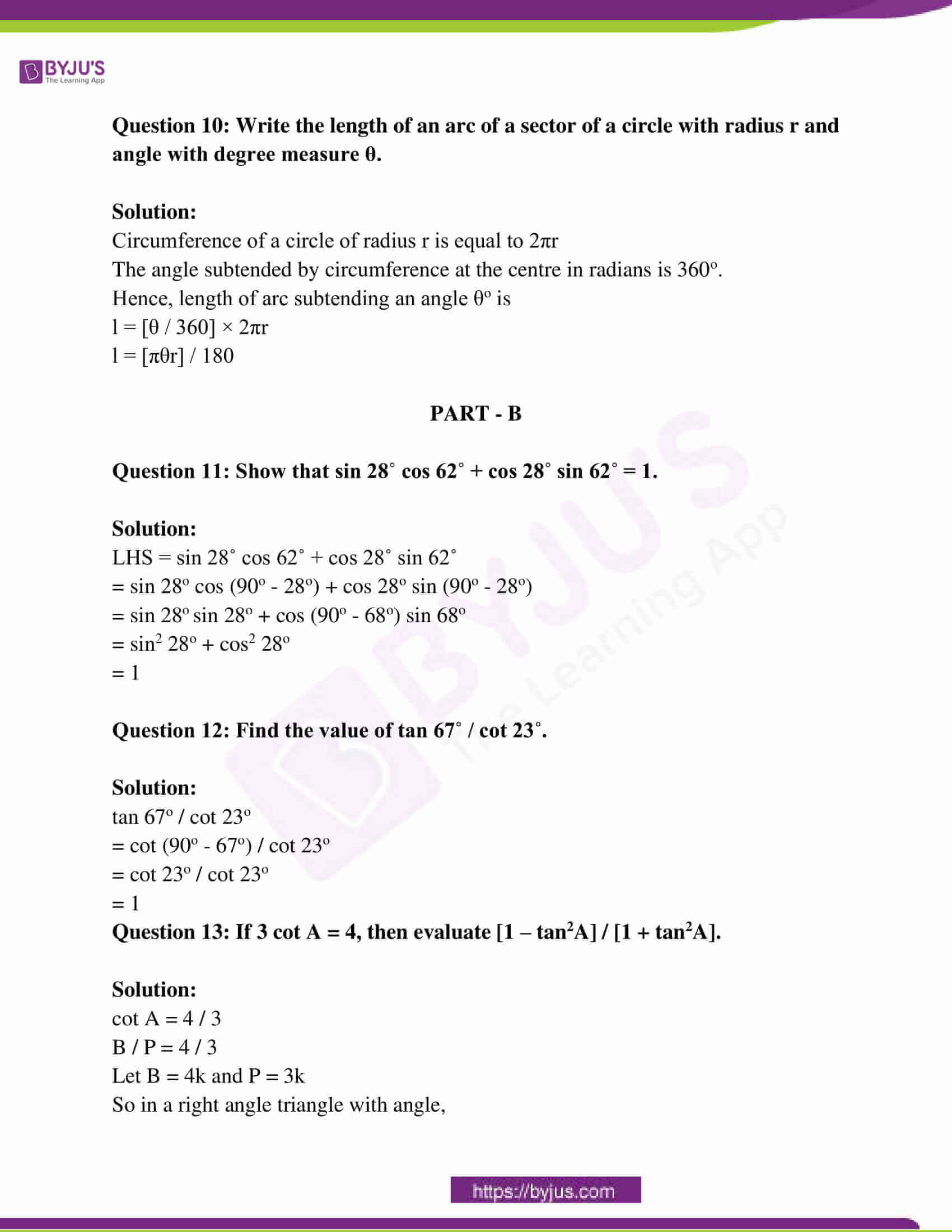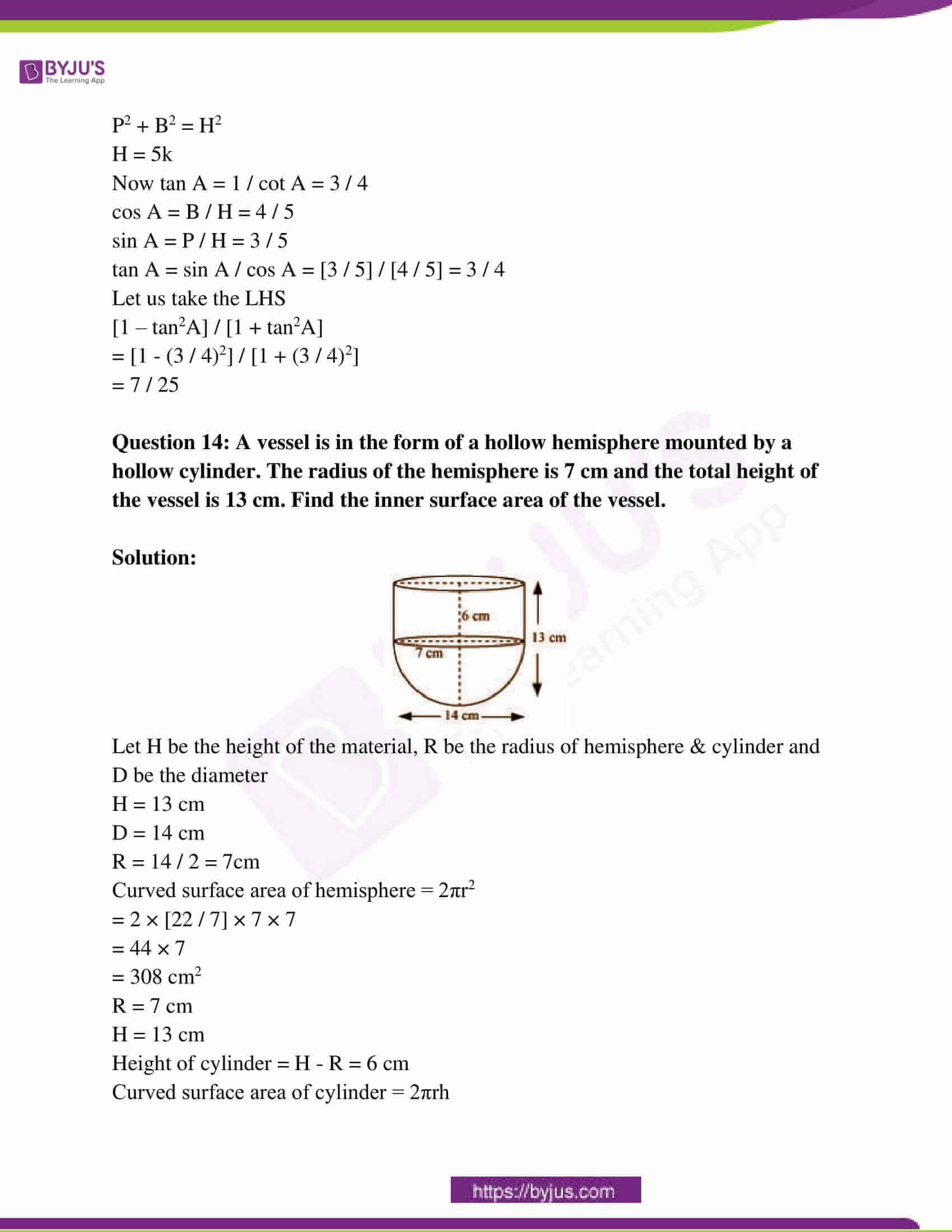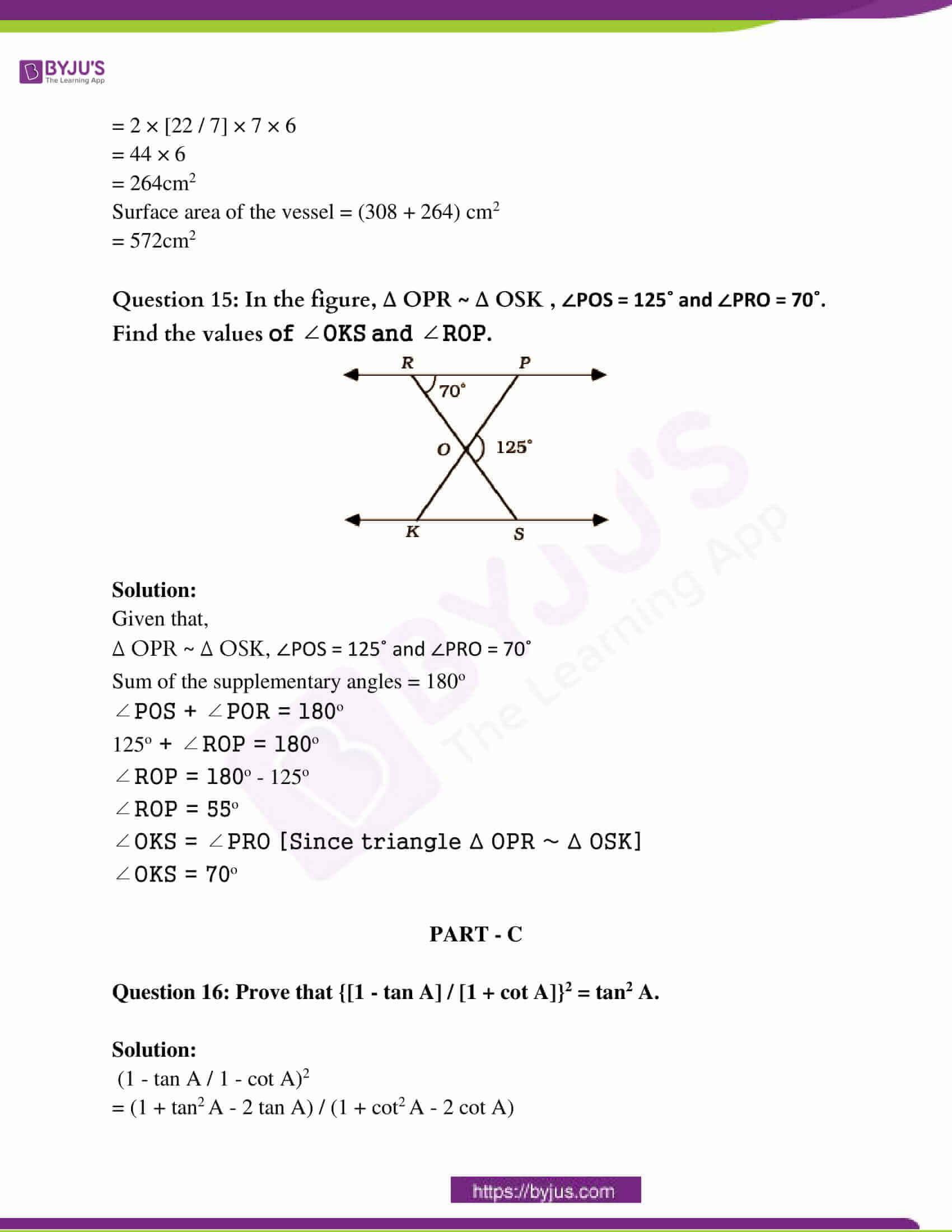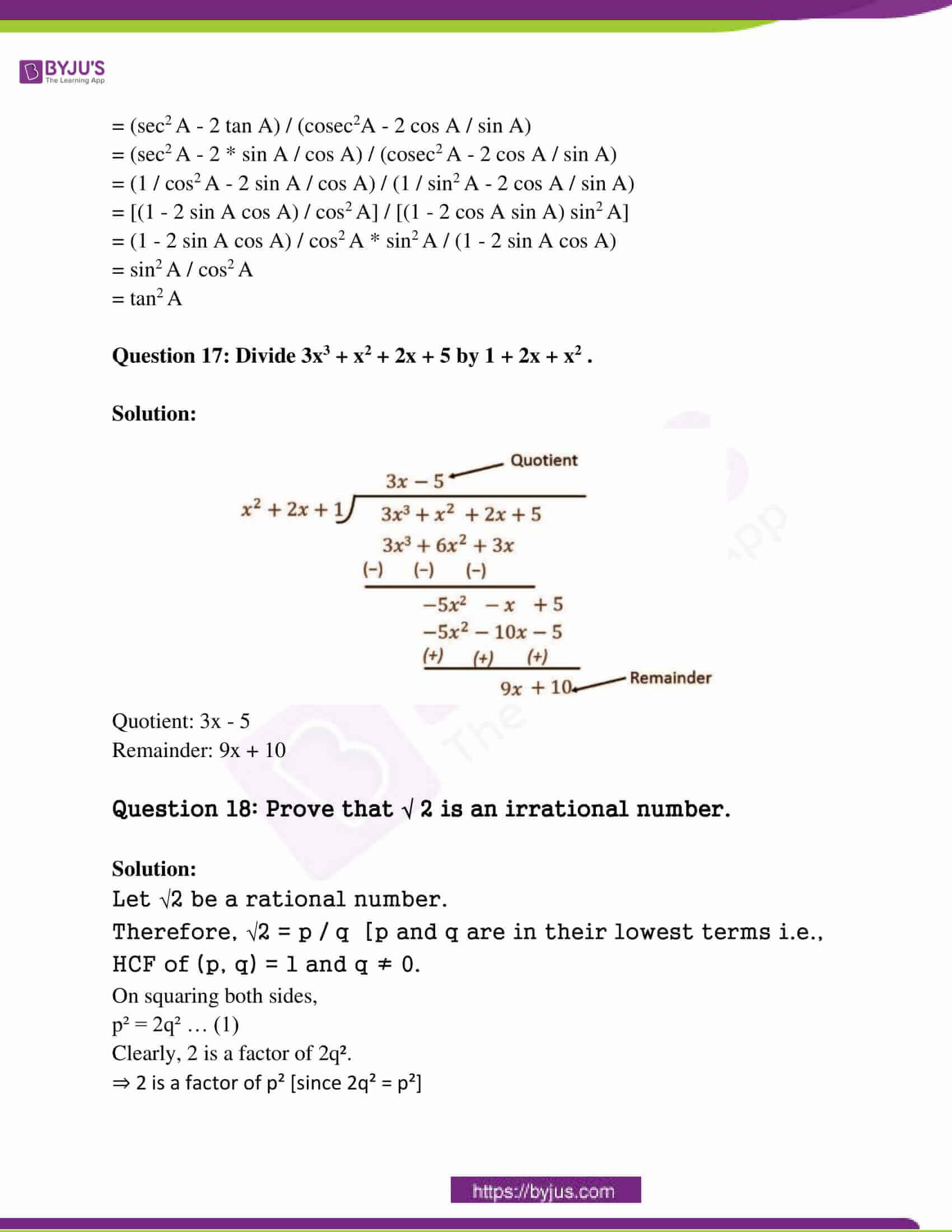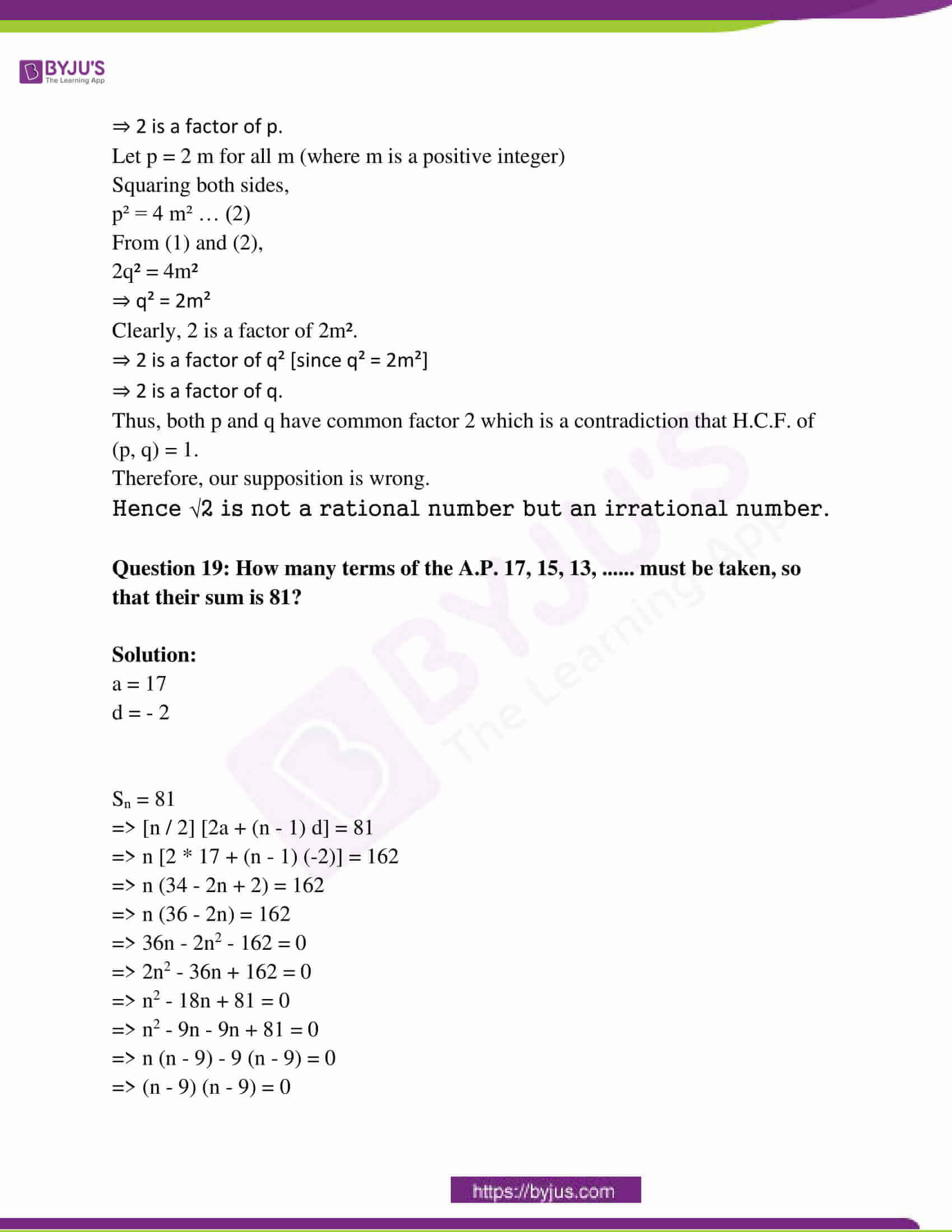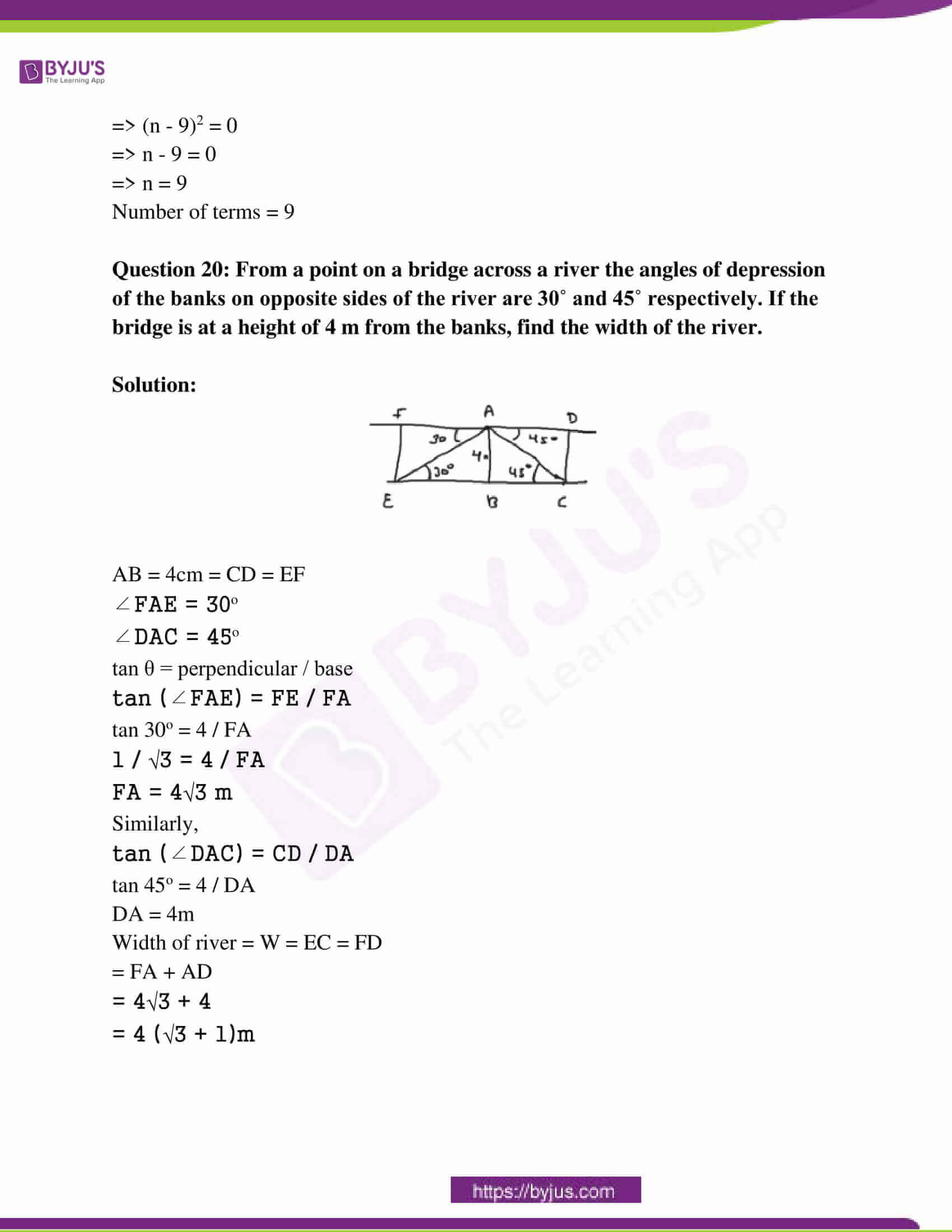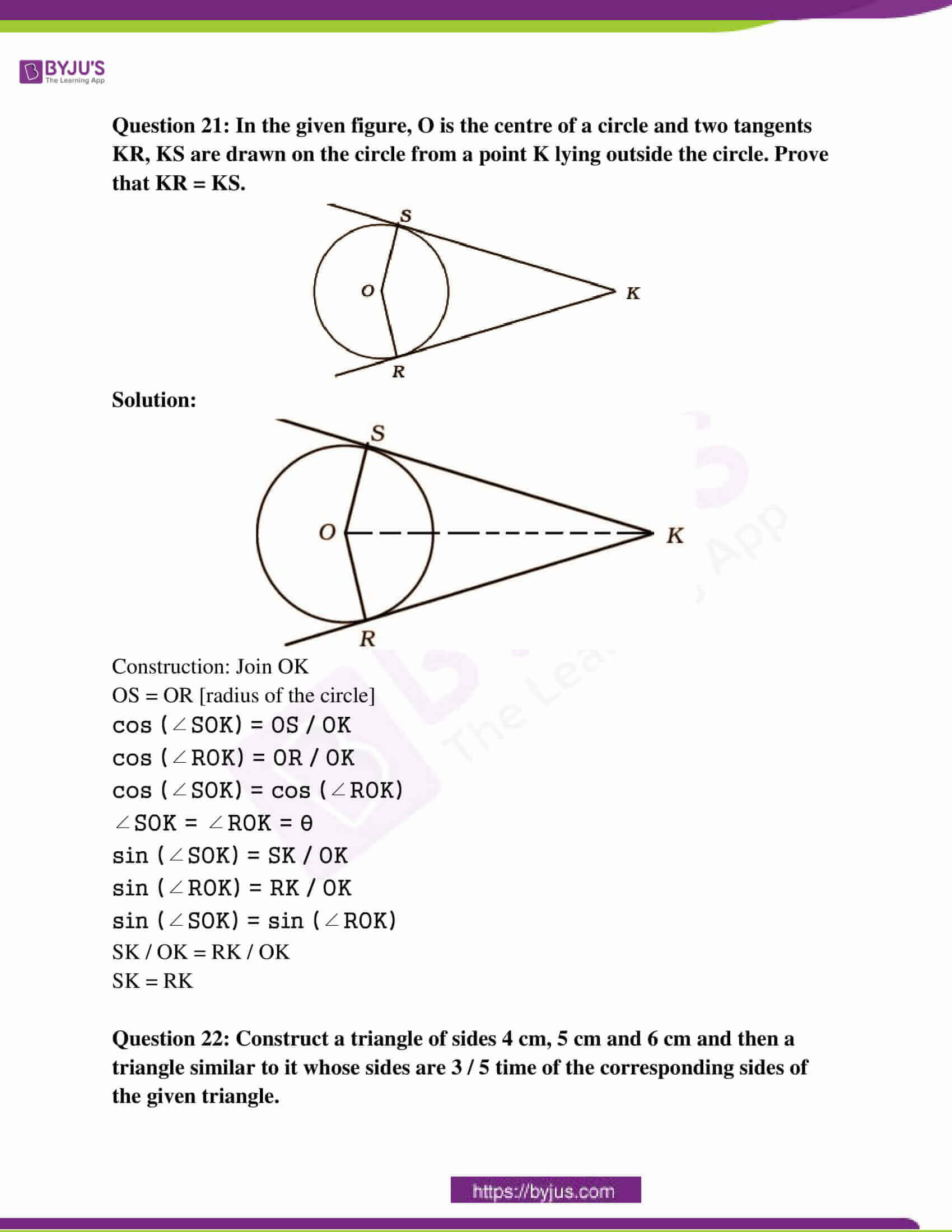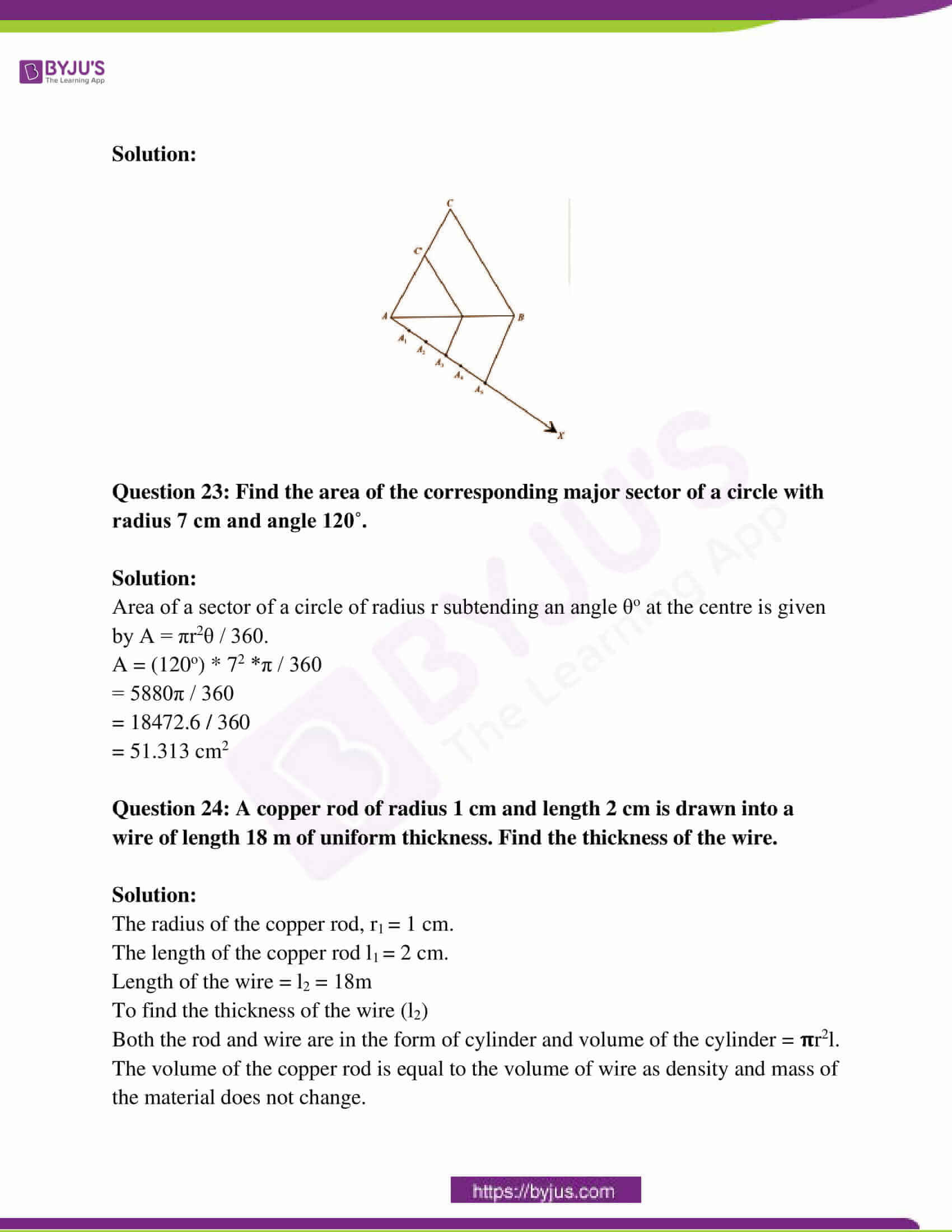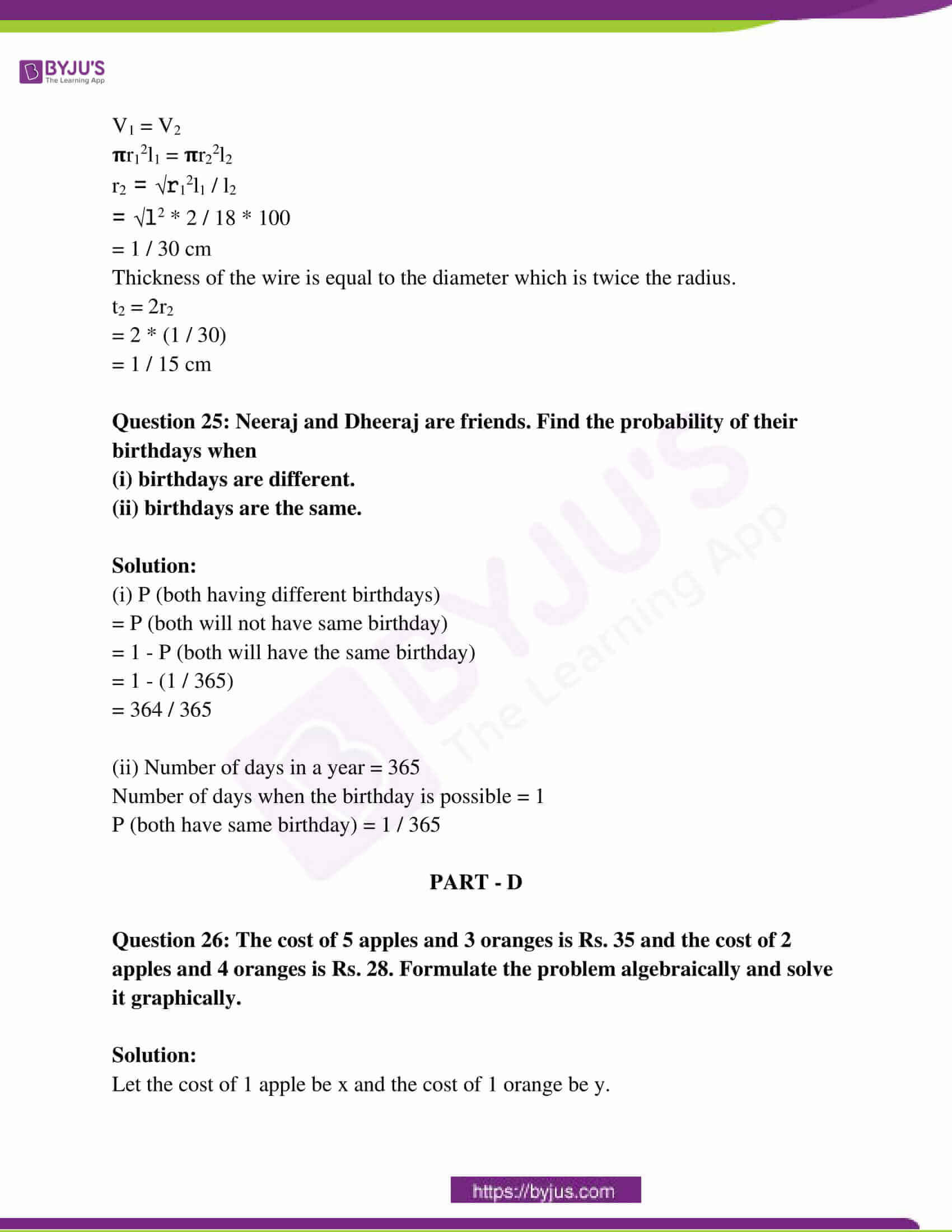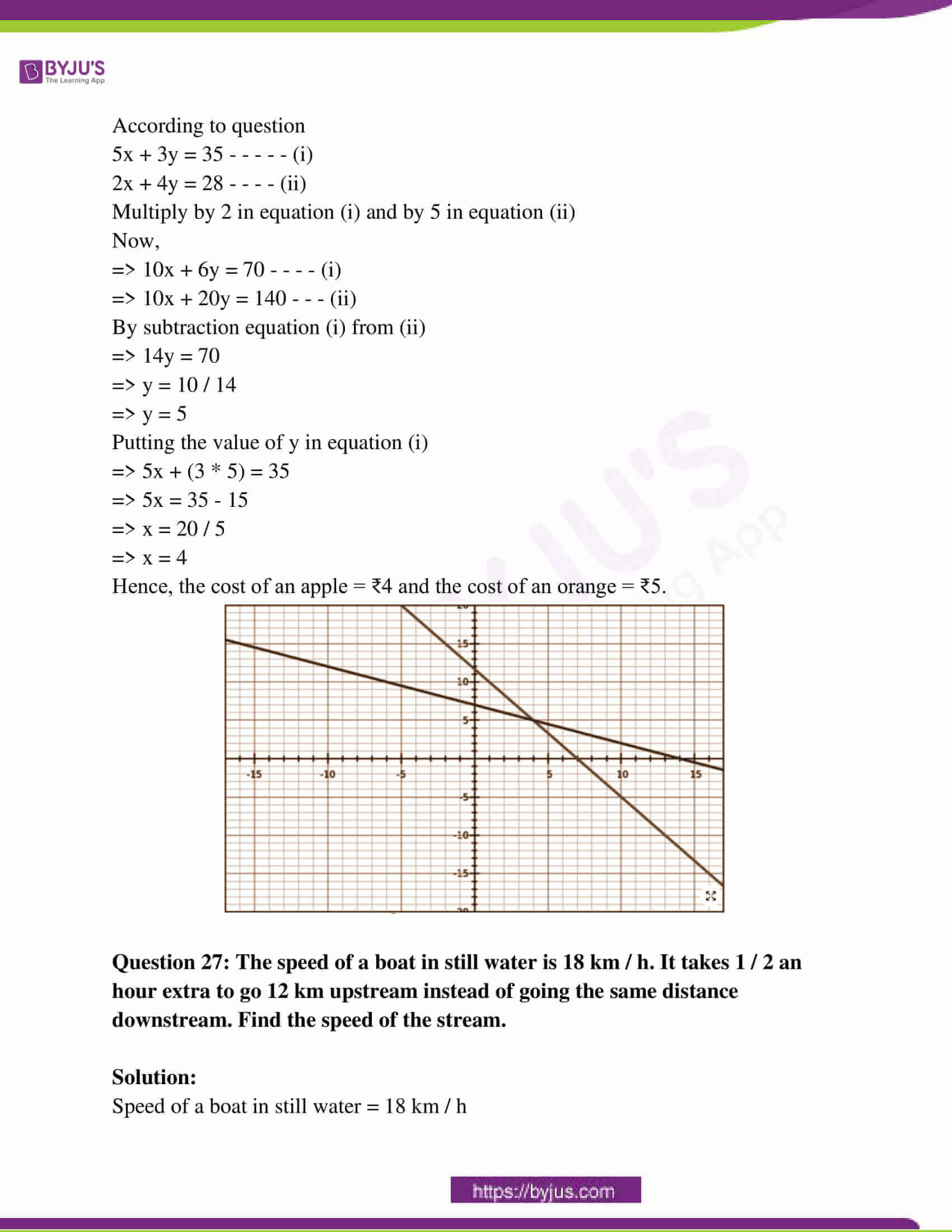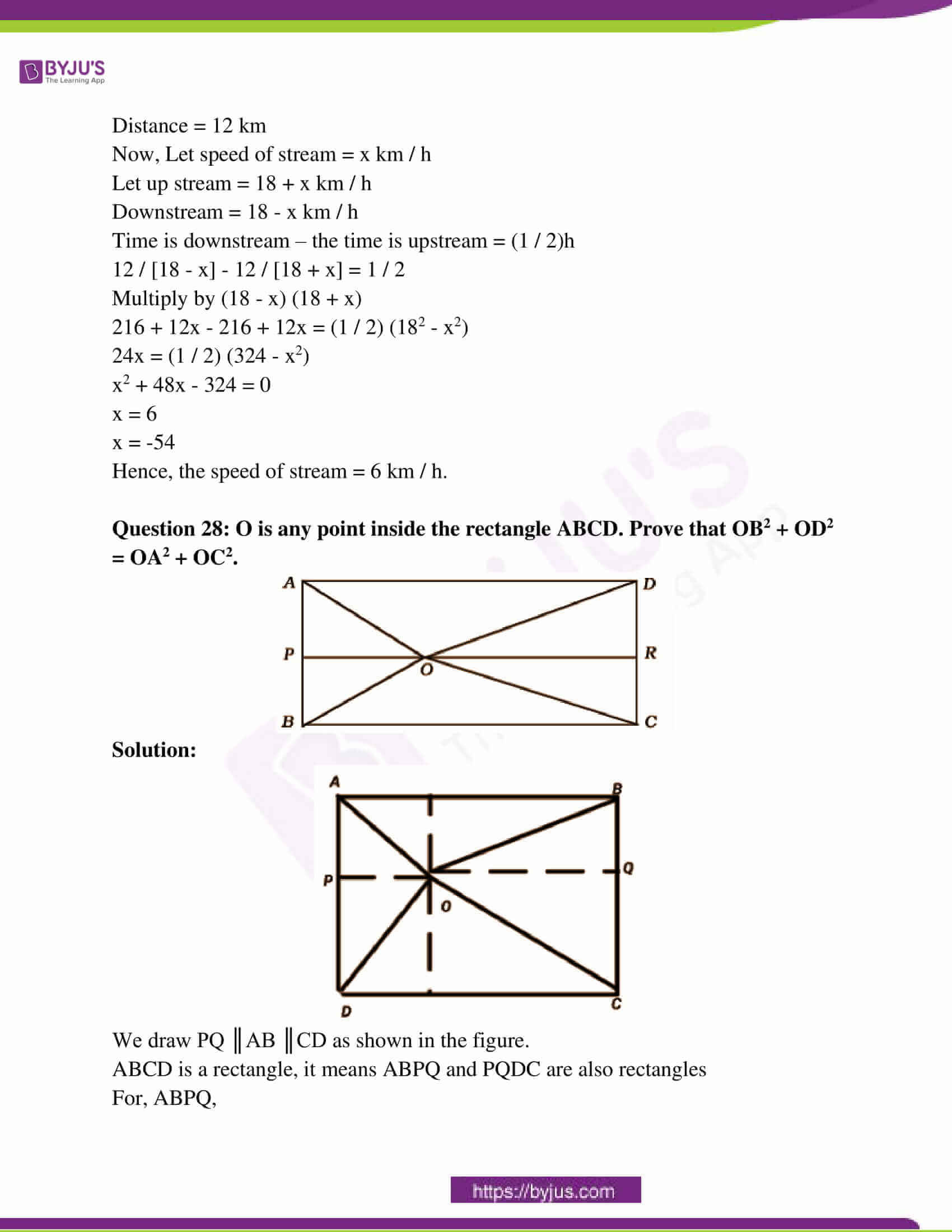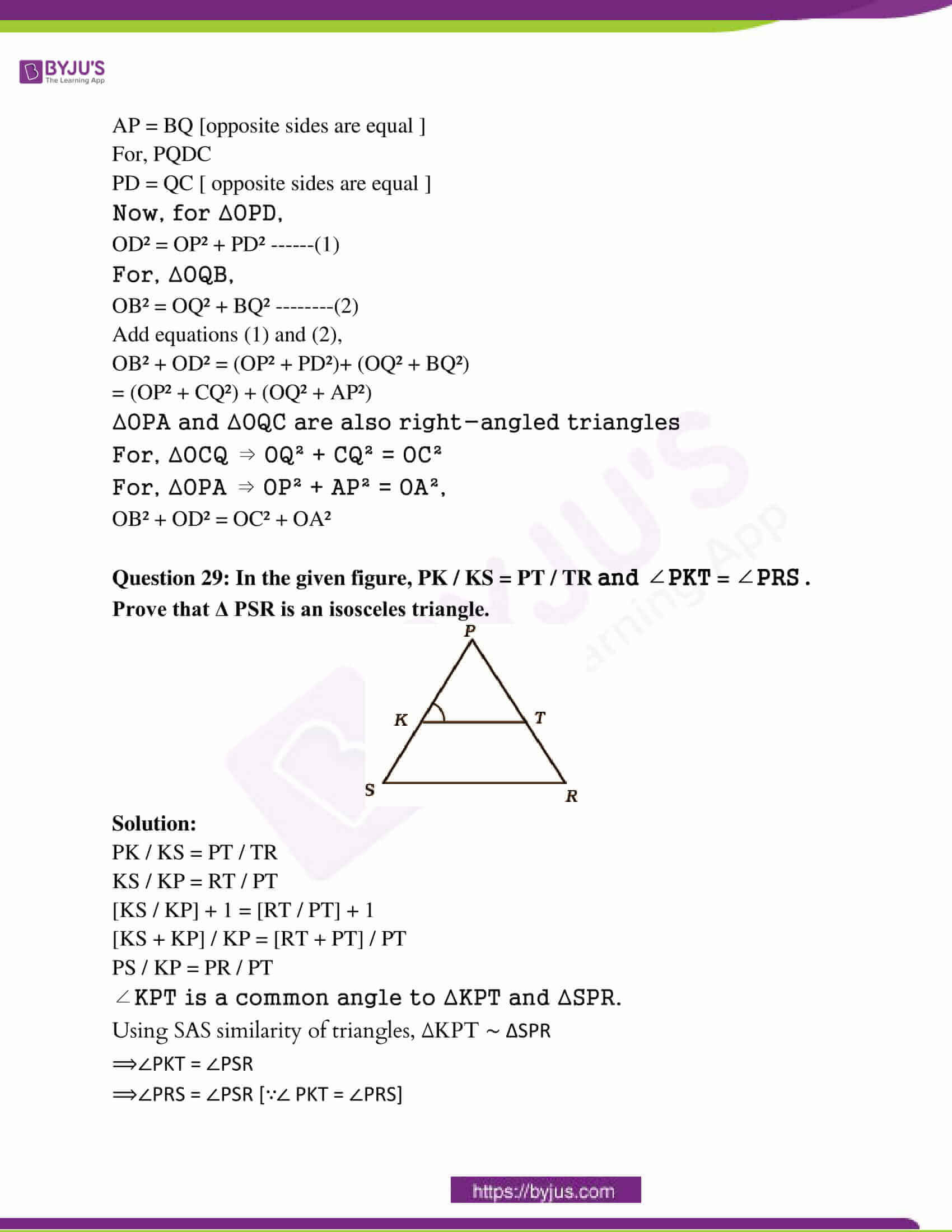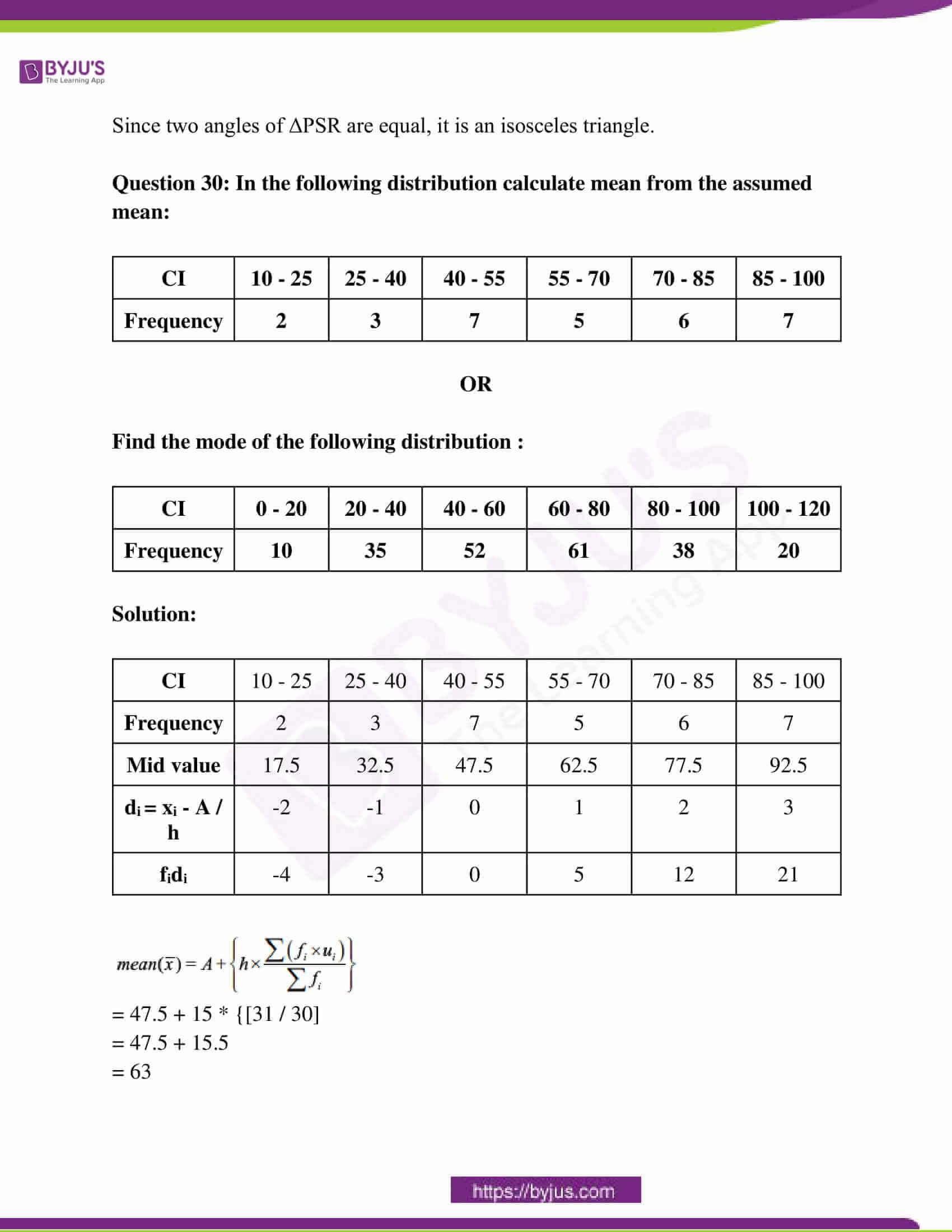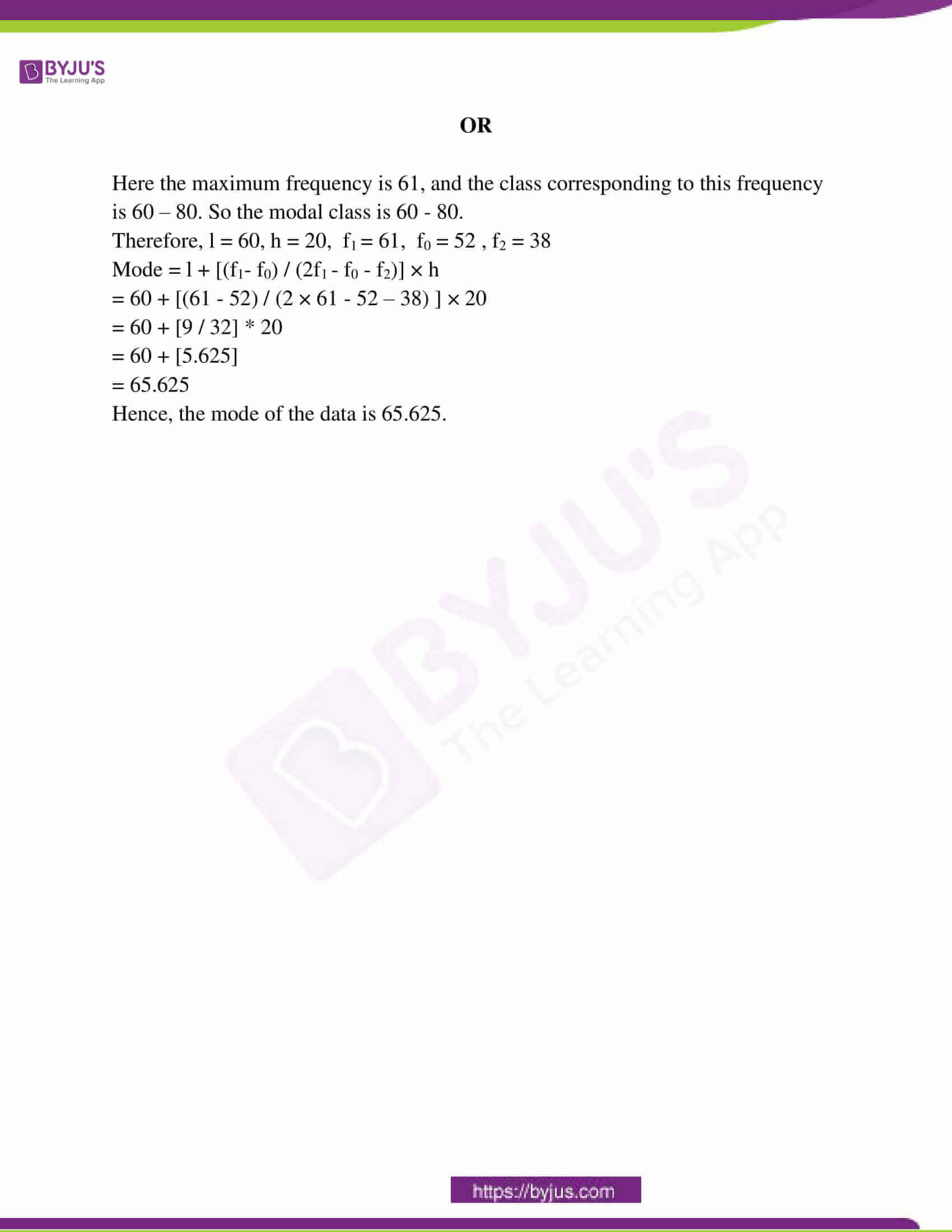### RBSE Class 10th Maths Question Paper With Solutions 2015

PART – A

Question 1: Write the common difference of the A.P. 7, 5, 3, 1, – 1, – 3, ……

Solution:

The given AP: 7, 5, 3, 1, – 1, – 3, ……

The first term a = 7

Common difference [d] = a2 – a1

= 5 – 7

= -2

Hence, the common difference is -2.

Question 2: Write the distance of the point (– 5, 4) from the x-axis.

Solution:

The distance of the point (-5, 4) from x-axis means the distance of the point (-5,4) from (-5,0).

Therefore, the required distance is given by distance formula

= √(x2 – x1)2 + (y2 – y1)2

= √(- 5 + 5)2 + (4 – 0)2

= √0 + 16

= √42

= 4

Question 3: Write the solution of the pair of linear equations 4x + 2y = 5 and x – 2y = 0.

Solution:

4x + 2y = 5 —- (1)

x – 2y = 0

x = 2y —- (2)

Put equation (2) in (1),

4 * (2y) + 2y = 5

10y = 5

y = 5 / 10

y = 1 / 2

Putting the value of y in (2),

x = 2y

x = 2 (1 / 2)

x = 1

Question 4: Find the HCF of 96 and 404 by the Prime Factorisation Method.

Solution: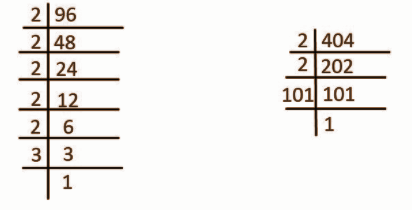96 = 2 × 2 × 2 × 2 × 2 × 3

404 = 2 × 2 × 101

HCF of 96 and 404 = Product of common prime factors

= 2 × 2

= 4

Question 5: One card is drawn from a well-shuffled deck of 52 cards. Calculate the probability that the card will not be an ace.

Solution:

The total number of favourable outcomes n (S) = 52.

Probability of getting an ace card = 4.

P (getting an ace card) = n(A) / n(S)

= 4 / 52

= 1 / 13

P (not getting an ace card) = 1 – P (getting an ace card)

= 1 – (1 / 13)

= 12 / 13

Question 6: If K ( 5, 4 ) is the midpoint of the line segment PQ and coordinates of Q are ( 2, 3 ), then find the coordinates of point P.

Solution:

Given that, PQ is a line segment with K its mid-point

Let, P ≡ (x1, y1) and Q ≡ (x2, y2)

The coordinates of Q are (2, 3) and that of mid-point K are (5, 4)

By the definition of midpoint,

x coordinates of midpoint = [x1 + x2] / 

y coordinates of midpoint = [y1 + y2] / 

5 = [x1 + 2] / 

10 = [x1 + 2]

10 – 2 = x1

x1 = 8

4 = [y1 + 3] / 

​8 = [y1 + 3]

8 – 3 = y1

y1 = 5

Hence, the coordinates of point P are (8, 5).

Question 7: If tangents RA and RB from a point R to a circle with centre O are inclined to each other at an angle of θ and ∠AOB = 40˚ then find the value of θ.

Solution: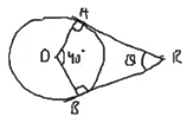∠OAR = 90o

∠OBR = 90o

In the quadrilateral OARB, the sum of the angles of a quadrilateral is 360o.

∠OAR + ∠OBR + ∠ARB + ∠AOB = 360o

90o + 90o + θ + 40o = 360o

220o + θ = 360o

θ = 360o – 220o

θ = 140o

Question 8: How many tangents can be constructed to any point on the circle of radius 4 cm?

Solution:

One tangent passes through a given point irrespective of its radius.

At a point on the circle, only one tangent can be drawn as it is perpendicular to the normal at the point.

Question 9: Find the circumference of a circle whose diameter is 14 cm.

Solution:

The radius will be half of the diameter.

The diameter of the circle = 14 cm

Radius of the circle = 14 / 2 = 7 cm

Circumference = 2Πr

= 2 * Π * 7

= 2 * (22 / 7) * 7

= 43.98 cm

Question 10: Write the length of an arc of a sector of a circle with radius r and angle with degree measure θ.

Solution:

Circumference of a circle of radius r is equal to 2πr

The angle subtended by circumference at the centre in radians is 360o.

Hence, length of arc subtending an angle θo is

l = [θ / 360] × 2πr

l = [πθr] / 180

PART – B

Question 11: Show that sin 28˚ cos 62˚ + cos 28˚ sin 62˚ = 1.

Solution:

LHS = sin 28˚ cos 62˚ + cos 28˚ sin 62˚

= sin 28o cos (90o – 28o) + cos 28o sin (90o – 28o)

= sin 28o sin 28o + cos (90o – 68o) sin 68o

= sin2 28o + cos2 28o

= 1

Question 12: Find the value of tan 67˚ / cot 23˚.

Solution:

tan 67o / cot 23o

= cot (90o – 67o) / cot 23o

= cot 23o / cot 23o

= 1

Question 13: If 3 cot A = 4, then evaluate [1 – tan2A] / [1 + tan2A].

Solution:

cot A = 4 / 3

B / P = 4 / 3

Let B = 4k and P = 3k

So in a right angle triangle with angle,

P2 + B2 = H2

H = 5k

Now tan A = 1 / cot A = 3 / 4

cos A = B / H = 4 / 5

sin A = P / H = 3 / 5

tan A = sin A / cos A = [3 / 5] / [4 / 5] = 3 / 4

Let us take the LHS

[1 – tan2A] / [1 + tan2A]

= [1 – (3 / 4)2] / [1 + (3 / 4)2]

= 7 / 25

Question 14: A vessel is in the form of a hollow hemisphere mounted by a hollow cylinder. The radius of the hemisphere is 7 cm and the total height of the vessel is 13 cm. Find the inner surface area of the vessel.

Solution: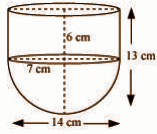Let H be the height of the material, R be the radius of hemisphere & cylinder and D be the diameter

H = 13 cm

D = 14 cm

R = 14 / 2 = 7cm

Curved surface area of hemisphere = 2πr2

= 2 × [22 / 7] × 7 × 7

= 44 × 7

= 308 cm2

R = 7 cm

H = 13 cm

Height of cylinder = H – R = 6 cm

Curved surface area of cylinder = 2πrh

= 2 × [22 / 7] × 7 × 6

= 44 × 6

= 264cm2

Surface area of the vessel = (308 + 264) cm2

= 572cm2

Question 15: In the figure, Δ OPR ~ Δ OSK , ∠POS = 125˚ and ∠PRO = 70˚. Find the values of ∠OKS and ∠ROP.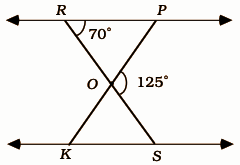Solution:

Given that,

Δ OPR ~ Δ OSK, ∠POS = 125˚ and ∠PRO = 70˚

Sum of the supplementary angles = 180o

∠POS + ∠POR = 180o

125o + ∠ROP = 180o

∠ROP = 180o – 125o

∠ROP = 55o

∠OKS = ∠PRO [Since triangle Δ OPR ~ Δ OSK]

∠OKS = 70o

PART – C

Question 16: Prove that {[1 – tan A] / [1 + cot A]}2 = tan2 A.

Solution:

(1 – tan A / 1 – cot A)2

= (1 + tan2 A – 2 tan A) / (1 + cot2 A – 2 cot A)

= (sec2 A – 2 tan A) / (cosec2A – 2 cos A / sin A)

= (sec2 A – 2 * sin A / cos A) / (cosec2 A – 2 cos A / sin A)

= (1 / cos2 A – 2 sin A / cos A) / (1 / sin2 A – 2 cos A / sin A)

= [(1 – 2 sin A cos A) / cos2 A] / [(1 – 2 cos A sin A) sin2 A]

= (1 – 2 sin A cos A) / cos2 A * sin2 A / (1 – 2 sin A cos A)

= sin2 A / cos2 A

= tan2 A

Question 17: Divide 3x3 + x2 + 2x + 5 by 1 + 2x + x2 .

Solution: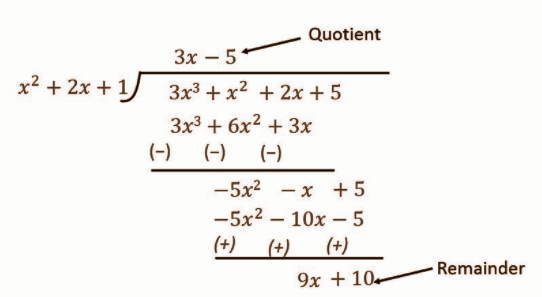Quotient: 3x – 5

Remainder: 9x + 10

Question 18: Prove that √ 2 is an irrational number.

Solution:

Let √2 be a rational number.

Therefore, √2 = p / q [p and q are in their lowest terms i.e., HCF of (p, q) = 1 and q ≠ 0.

On squaring both sides,

p² = 2q² … (1)

Clearly, 2 is a factor of 2q².

⇒ 2 is a factor of p² [since 2q² = p²]

⇒ 2 is a factor of p.

Let p = 2 m for all m (where m is a positive integer)

Squaring both sides,

p² = 4 m² … (2)

From (1) and (2),

2q² = 4m²

⇒ q² = 2m²

Clearly, 2 is a factor of 2m².

⇒ 2 is a factor of q² [since q² = 2m²]

⇒ 2 is a factor of q.

Thus, both p and q have common factor 2 which is a contradiction that H.C.F. of (p, q) = 1.

Therefore, our supposition is wrong.

Hence √2 is not a rational number but an irrational number.

Question 19: How many terms of the A.P. 17, 15, 13, …… must be taken, so that their sum is 81?

Solution:

a = 17

d = – 2

Sn = 81

=> [n / 2] [2a + (n – 1) d] = 81

=> n [2 * 17 + (n – 1) (-2)] = 162

=> n (34 – 2n + 2) = 162

=> n (36 – 2n) = 162

=> 36n – 2n2 – 162 = 0

=> 2n2 – 36n + 162 = 0

=> n2 – 18n + 81 = 0

=> n2 – 9n – 9n + 81 = 0

=> n (n – 9) – 9 (n – 9) = 0

=> (n – 9) (n – 9) = 0

=> (n – 9)2 = 0

=> n – 9 = 0

=> n = 9

Number of terms = 9

Question 20: From a point on a bridge across a river the angles of depression of the banks on opposite sides of the river are 30˚ and 45˚, respectively. If the bridge is at a height of 4 m from the banks, find the width of the river.

Solution: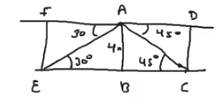AB = 4cm = CD = EF

∠FAE = 30o

∠DAC = 45o

tan θ = perpendicular / base

tan (∠FAE) = FE / FA

tan 30o = 4 / FA

1 / √3 = 4 / FA

FA = 4√3 m

Similarly,

tan (∠DAC) = CD / DA

tan 45o = 4 / DA

DA = 4m

Width of river = W = EC = FD

= 4√3 + 4

= 4 (√3 + 1)m

Question 21: In the given figure, O is the centre of a circle and two tangents KR, KS are drawn on the circle from a point K lying outside the circle. Prove that KR = KS.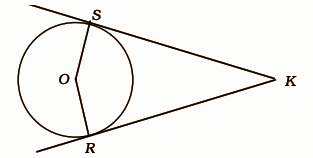Solution: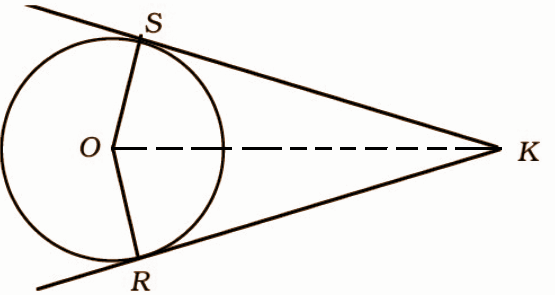Construction: Join OK

OS = OR [radius of the circle]

cos (∠SOK) = OS / OK

cos (∠ROK) = OR / OK

cos (∠SOK) = cos (∠ROK)

∠SOK = ∠ROK = θ

sin (∠SOK) = SK / OK

sin (∠ROK) = RK / OK

sin (∠SOK) = sin (∠ROK)

SK / OK = RK / OK

SK = RK

Question 22: Construct a triangle of sides 4 cm, 5 cm and 6 cm and then a triangle similar to it whose sides are 3 / 5 time of the corresponding sides of the given triangle.

Solution: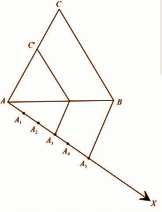Question 23: Find the area of the corresponding major sector of a circle with radius 7 cm and angle 120˚.

Solution:

Area of a sector of a circle of radius r subtending an angle θo at the centre is given by A = πr2θ / 360.

A = (120o) * 72 *π / 360

= 5880π / 360

= 18472.6 / 360

= 51.313 cm2

Question 24: A copper rod of radius 1 cm and length 2 cm is drawn into a wire of length 18 m of uniform thickness. Find the thickness of the wire.

Solution:

The radius of the copper rod, r1 = 1 cm.

The length of the copper rod l1 = 2 cm.

Length of the wire = l2 = 18m

To find the thickness of the wire (l2)

Both the rod and wire are in the form of cylinder and volume of the cylinder = 𝛑r2l.

The volume of the copper rod is equal to the volume of wire as density and mass of the material does not change.

V1 = V2

𝛑r12l1 = 𝛑r22l2

r2 = √r12l1 / l2

= √12 * 2 / 18 * 100

= 1 / 30 cm

Thickness of the wire is equal to the diameter which is twice the radius.

t2 = 2r2

= 2 * (1 / 30)

= 1 / 15 cm

Question 25: Neeraj and Dheeraj are friends. Find the probability of their birthdays when

(i) birthdays are different.

(ii) birthdays are the same.

Solution:

(i) P (both having different birthdays)

= P (both will not have same birthday)

= 1 – P (both will have the same birthday)

= 1 – (1 / 365)

= 364 / 365

(ii) Number of days in a year = 365

Number of days when the birthday is possible = 1

P (both have same birthday) = 1 / 365

PART – D

Question 26: The cost of 5 apples and 3 oranges is Rs. 35 and the cost of 2 apples and 4 oranges is Rs. 28. Formulate the problem algebraically and solve it graphically.

Solution:

Let the cost of 1 apple be x and the cost of 1 orange be y.

According to question

5x + 3y = 35 – – – – – (i)

2x + 4y = 28 – – – – (ii)

Multiply by 2 in equation (i) and by 5 in equation (ii)

Now,

=> 10x + 6y = 70 – – – – (i)

=> 10x + 20y = 140 – – – (ii)

By subtraction equation (i) from (ii)

=> 14y = 70

=> y = 10 / 14

=> y = 5

Putting the value of y in equation (i)

=> 5x + (3 * 5) = 35

=> 5x = 35 – 15

=> x = 20 / 5

=> x = 4

Hence, the cost of an apple = ₹4 and the cost of an orange = ₹5.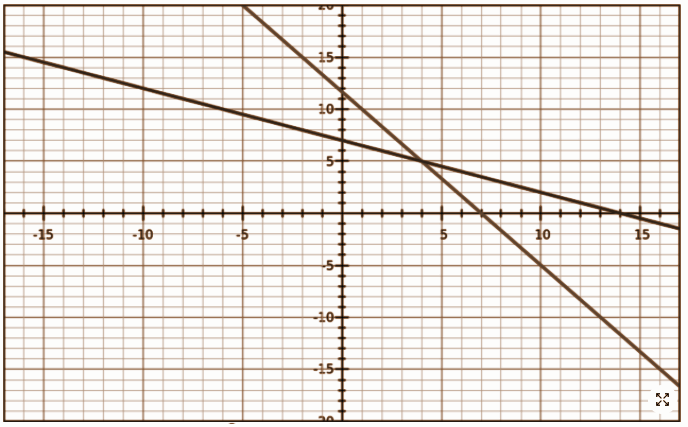Question 27: The speed of a boat in still water is 18 km / h. It takes 1 / 2 an hour extra to go 12 km upstream instead of going the same distance downstream. Find the speed of the stream.

Solution:

Speed of a boat in still water = 18 km / h

Distance = 12 km

Now, Let speed of stream = x km / h

Let up stream = 18 + x km / h

Downstream = 18 – x km / h

Time is downstream – the time is upstream = (1 / 2)h

12 / [18 – x] – 12 / [18 + x] = 1 / 2

Multiply by (18 – x) (18 + x)

216 + 12x – 216 + 12x = (1 / 2) (182 – x2)

24x = (1 / 2) (324 – x2)

x2 + 48x – 324 = 0

x = 6

x = -54

Hence, the speed of stream = 6 km / h.

Question 28: O is any point inside the rectangle ABCD. Prove that OB2 + OD2 = OA2 + OC2.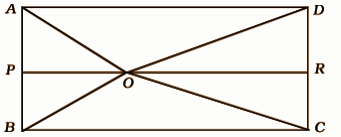Solution: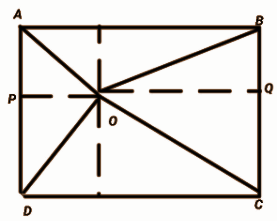We draw PQ ║AB ║CD as shown in the figure.

ABCD is a rectangle, it means ABPQ and PQDC are also rectangles

For, ABPQ,

AP = BQ [opposite sides are equal ]

For, PQDC

PD = QC [ opposite sides are equal ]

Now, for ∆OPD,

OD² = OP² + PD² ——(1)

For, ∆OQB,

OB² = OQ² + BQ² ——–(2)

OB² + OD² = (OP² + PD²)+ (OQ² + BQ²)

= (OP² + CQ²) + (OQ² + AP²)

∆OPA and ∆OQC are also right-angled triangles

For, ∆OCQ ⇒ OQ² + CQ² = OC²

For, ∆OPA ⇒ OP² + AP² = OA²,

OB² + OD² = OC² + OA²

Question 29: In the given figure, PK / KS = PT / TR and ∠PKT = ∠PRS . Prove that Δ PSR is an isosceles triangle.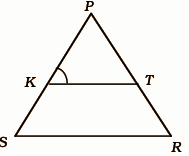Solution:

PK / KS = PT / TR

KS / KP = RT / PT

[KS / KP] + 1 = [RT / PT] + 1

[KS + KP] / KP = [RT + PT] / PT

PS / KP = PR / PT

∠KPT is a common angle to ΔKPT and ΔSPR.

Using SAS similarity of triangles, ΔKPT ∼ ΔSPR

⟹∠PKT = ∠PSR

⟹∠PRS = ∠PSR [∵∠ PKT = ∠PRS]

Since two angles of ΔPSR are equal, it is an isosceles triangle.

Question 30: In the following distribution calculate mean from the assumed mean:

 CI 10 – 25 25 – 40 40 – 55 55 – 70 70 – 85 85 – 100 Frequency 2 3 7 5 6 7

OR

Find the mode of the following distribution :

 CI 0 – 20 20 – 40 40 – 60 60 – 80 80 – 100 100 – 120 Frequency 10 35 52 61 38 20

Solution:

 CI 10 – 25 25 – 40 40 – 55 55 – 70 70 – 85 85 – 100 Frequency 2 3 7 5 6 7 Mid value 17.5 32.5 47.5 62.5 77.5 92.5 di = xi – A / h -2 -1 0 1 2 3 fidi -4 -3 0 5 12 21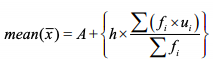= 47.5 + 15 * {[31 / 30]

= 47.5 + 15.5

= 63

OR

Here the maximum frequency is 61, and the class corresponding to this frequency is 60 – 80. So the modal class is 60 – 80.

Therefore, l = 60, h = 20, f1 = 61, f0 = 52 , f2 = 38

Mode = l + [(f1– f0) / (2f1 – f0 – f2)] × h

= 60 + [(61 – 52) / (2 × 61 – 52 – 38) ] × 20

= 60 + [9 / 32] * 20

= 60 + [5.625]

= 65.625

Hence, the mode of the data is 65.625.